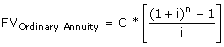# Lesson 2 - Value of Money - Part 7As we can see from the chart we need a few variables in order to calculate how much savings will be required to achieve our Goals. We have provided an Excel file which can be downloaded with all of the equations already built in.

We will go over some variables that are required. First we must know how long we have before we need the money for whatever Goal it might be. In our example, we will retire in 34 years. This is how long we have to save, and so on. In the next column we have our Inflation Rate. Since Our Goals take place in the future, we must assume that things will cost more in the future. I’m certain that \$100 dollars today will not be able to buy the same amount of goods 40 years from now.

Therefore, we must adjust the cost of our Goals for how much inflation they will incur over the years. Luckily calculating the amount of inflation that will affect our savings requirements is the same as calculating an Ordinary Annuity. Reminder an Ordinary Annuity is calculated by:C = Cash Amount
i = Interest Rate
n = number of payments